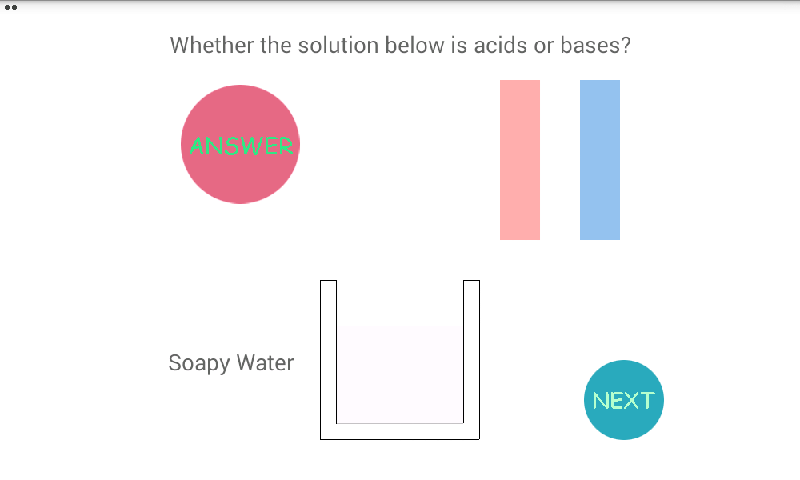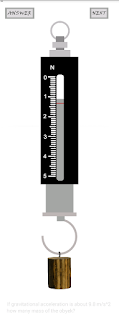## Posts

Showing posts from February, 2016

### Learn How Determine pH of Solution with pH Paper GamespH Paper Games is simple app that simulate how to determine pH value of the solution, based on changing the pH paper color. Basically if pH paper dipped into the solution, the paper will be changing the color appropriate the pH value itselft. Please try to guess the pH value at every solution by matching the changing color with the reference pH color beside it.

here video preview of how to use this app:

You can download this app free at link below: https://play.google.com/store/apps/details?id=com.priantos.phpaper

### Litmus Paper : Simple App that Simulate How to Determine Acids or BasesLitmus Paper is simple simulation how to determine acid or base of the solution, based on changing the litmus color. Basically, if the solution is acidic, the solution can redden blue litmus. And if the solution is base, the solution can be blueing red litmus. Based on the condition, then if the litmus paper dipped into the solution, we will be able to identify whether a solution is acidic or bases.

Here few screenshot and short video tutorial how to use this App:

You can download it free at link below:

https://play.google.com/store/apps/details?id=com.priantos.litmuspaper

### Simple Spring Scale Simulator and GamesSpring Scale is a simple game that designed to simulate the technique of how to read of do measurement with the Spring Scale. This app is one of Computational Lab of Department Physics, UB productivity to increase the passion of student learning about physics in Elementary Physics Lab. You can try to measure how many mass at every object and interact with the scale.This is given the random mass for every section, and also you may find different object that to be measure.

The basic concept of this simulation, are based on spring forced that mathematically writed as W=-ky, where W is weight of object that to be measure (N), k is spring constant (N/m), and y is the deviation of spring (m). So if W=mg, with m is mass of the object (kg) and g is gravitational acceleration (m/s2).

The scale result of measurement shown that weight of object is about 1.325 Newton. With gravitational acceleration 9.8 m/s2, and based on equation W=mg, We can calculate the mass of object is about 0.1352 kg.

here…